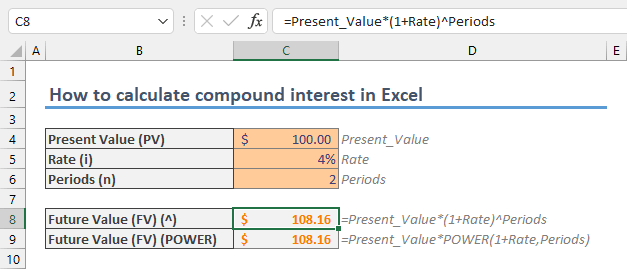In this guide, we’re going to show you how to calculate compound interest in Excel.

## Compound interest

Compound interest means "interest on the interest", which defines the interest calculation based on both the initial principal and the accumulated interest from previous periods.

For example, if you get interest on \$100 at 4% for the year, you will have \$104 (\$100 * 1.04) at the end of the year. If you re-invest your entire \$104 again, you will see \$108.16 (\$104 * 1.04) in your account the year after.

Here is comparison between simple interest and compound interest by years:

 Time Simple Interest@ 4% Compound Interest@ 4% Start 100 100 1 year \$104.00 \$104.00 2 years \$108.00 \$108.16 5 years \$120.00 \$121.67

## Calculating compound interest

You can calculate compound interest using the formula below or Excel's FV function.

### The formulaWhere:

• FV: The future value of the investment. This is the amount you will get at the end.
• PV: The present value of the investment. This is your initial value.
• i: The interest rate by period.
• n: The number of periods.

For example; our \$100 investment becomes 100 * (1 + 0.04)² = \$108.16 at 4% after 2 years.

There is only one tricky part to implement this formula in Excel: exponential calculation. You can use either the caret (^) character or the POWER function to calculate the result of (1 + i) to the number of periods.

=Present_Value*(1+Rate)^Periods=Present_Value*POWER(1+Rate,Periods)### Excel Formula

The FV function can return the future value of a loan or an investment, based on given constant payments and interest rate.

FV(rate, nper, pmt, [pv], [type])

 rate The interest rate. nper The number of periods. pmt The constant payments during investment or loan. [pv] Optional. The present value of the investment. [type] Optional. When the payments are due.0 = end of period. (Default)1 = beginning of period.

The rate, nper, and pv arguments are required. The pmt in this example is 0 since there are no payments. The type argument is also related with payments, so feel free to omit it from the function.

One important thing here is the sign of the pv value. Typically, the present value (pv) should be a negative value, because you give it as part of an investment or deal.

Based on the information above, a generic syntax will be like below.

=FV(Rate,Periods,0,-Present_Value)No matter what approach you use, make sure that the period type and interest rates match. For example, if you want to calculate monthly interest at an annual rate, divide the rate by 12.